You may also likeInequalities

A bag contains 12 marbles. There are more red than green but green and blue together exceed the reds. The total of yellow and green marbles is more than the total of red and blue. How many of each colour there are in the bag?What's it Worth?

There are lots of different methods to find out what the shapes are worth - how many can you find?Turnips

Baldrick could buy 6 parsnips and 7 turnips, or 8 parsnips and 4 turnips. How many parsnips could he buy?

Magic 7

Age 11 to 14 Short Challenge Level:

7 + 8 + 9 + 10 + 11 + 12 + 13 + 14 + 15 = 99 = sum of all 3 rows
$\therefore$ sum of each row is 33

Writing the numbers in terms of $n$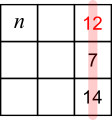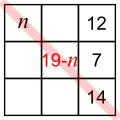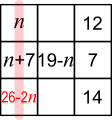\begin{align}26-2n\ \ +\ \ 19-n\ \ +\ \ 12\ \ &=33\\ 26+19 + 12-33&=3n\\ 24&=3n\\ 8&=n\end{align}

Writing the numbers in terms of $x$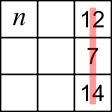7 + 14 = 21, 12 is missing to make 33Say $n$ + 12 + $x$ + 12 = 33,

then $n$ + 14 + ($x-$2) = 33Same idea gives $x-$4 at the bottom

$\therefore$ 3$x$-6=33, so $x$ = 13

And $n$ + $x$ + 12 = 33 $\Rightarrow n$ + 13 + 12 = 33 $\Rightarrow n$ = 8

Using reasoning and not algebra7 + 14 = 21, 12 is missing to make 33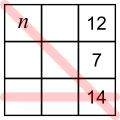33 $-$ 14 = 19
19 = 7 + 12
19 = 8 + 11
19 = 9 + 10
no more options so these 4 numbers are 8, 9, 10, 11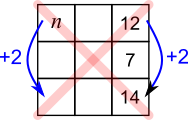$n$ + middle + 14 = bottom + middle + 12

difference between $n$ and bottom = difference between 12 and 14

This gives 2 options: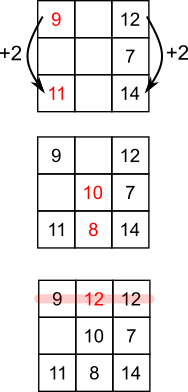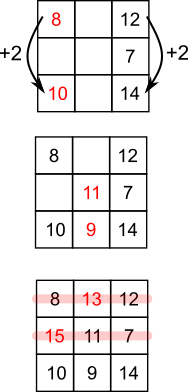This problem is taken from the UKMT Mathematical Challenges.
You can find more short problems, arranged by curriculum topic, in our short problems collection.